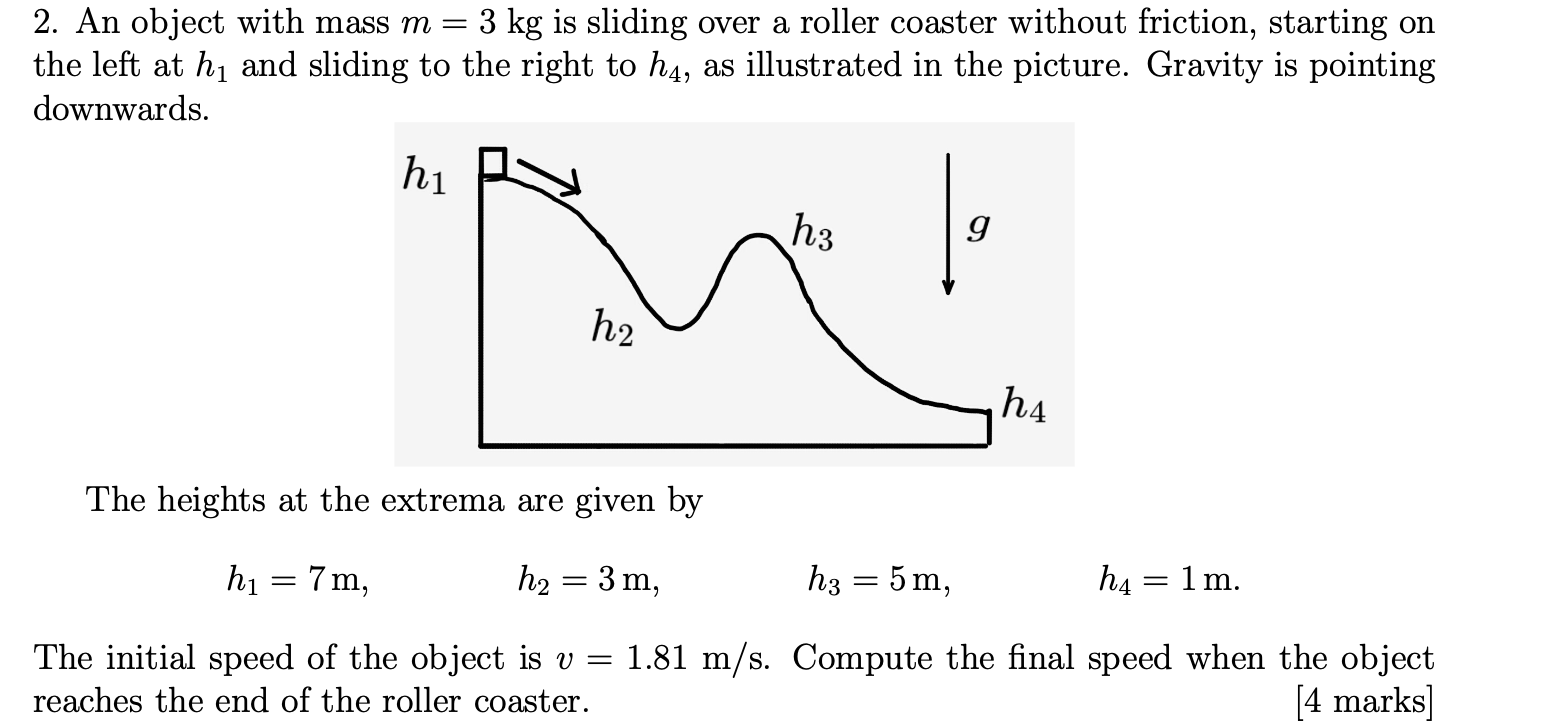Home / Expert Answers / Physics / 2-an-object-with-mass-m-3-mathrm-kg-is-sliding-over-a-roller-coaste-pa911

# (Solved): 2. An object with mass $$m=3 \mathrm{~kg}$$ is sliding over a roller coaste ...???????

2. An object with mass $$m=3 \mathrm{~kg}$$ is sliding over a roller coaster without friction, starting on the left at $$h_{1}$$ and sliding to the right to $$h_{4}$$, as illustrated in the picture. Gravity is pointing downwards. The heights at the extrema are given by $h_{1}=7 \mathrm{~m}, \quad h_{2}=3 \mathrm{~m}, \quad h_{3}=5 \mathrm{~m}, \quad h_{4}=1 \mathrm{~m}$ The initial speed of the object is $$v=1.81 \mathrm{~m} / \mathrm{s}$$. Compute the final speed when the object reaches the end of the roller coaster. [4 marks]

We have an Answer from Expert